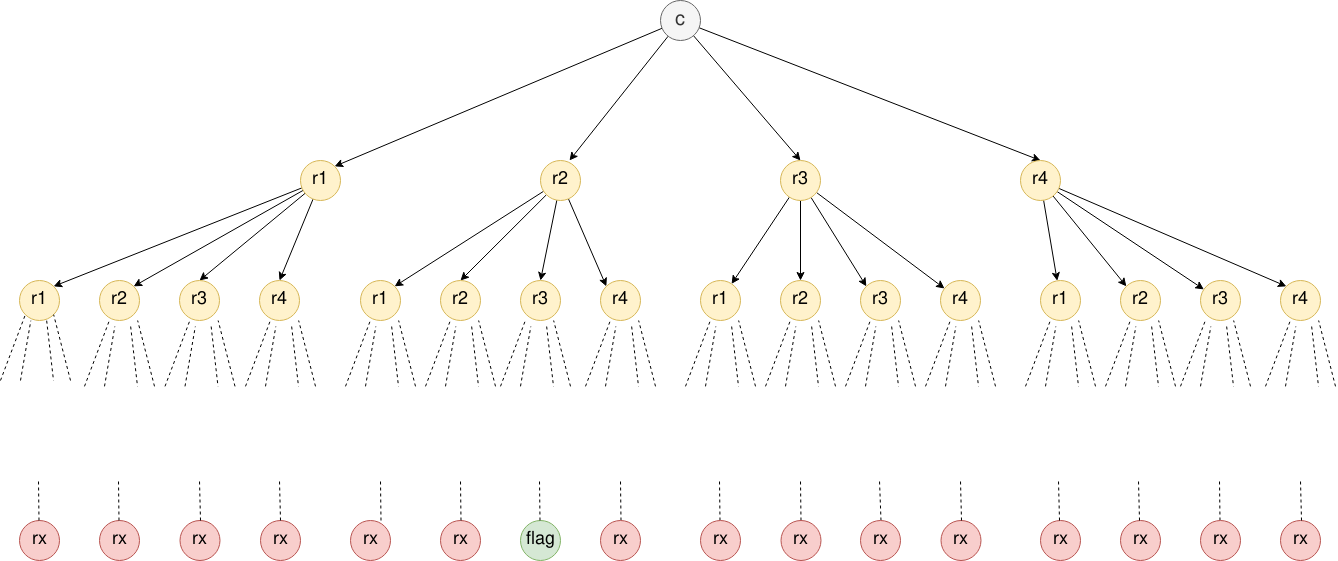# Writeup: HackCon 2019 - AgainAndAgainAndAgain

## Information

• category : crypto
• points : 467

## Description

Someone was thinking encrypting again and again helps, prove them wrong.

c = 196353764385075548782571270052469419021844481625366305056739966550926484027148967165867708531585849658610359148759560853

File : q1.py

## Writeup

Content of `q1.py` :

We have : `n = p * q, p, q, c`. To encrypt a message `m` the script does : `(m ^ 2) % n = c`.

The encryption remembers RSA, however in this case we have `e = 2` which is not a possible exponent in RSA because `gcd(2, phi(n)) = 2` and so the inverse of `e` in `phi(n)` is not possible. This cryptosystem with exponent 2 is the Rabin cryptosystem.

Decrypting a ciphertext `c` we get 4 different results. To decrypt we need to:

• Compute `mp = c ^ (1/4 * (p + 1)) mod p`
• Compute `mq = c ^ (1/4 * (q + 1)) mod q`
• Both can be expressed as :
• `mp = sqrt(c) mod p`
• `mq = sqrt(c) mod q`
• Use Tonelli-Shanks algorithm to compute both of them.
• Use Extended Euclidean algorithm to find `yp` and `yq` s.t. `yp * p + yq * q = gcd(p, q) = 1`
• Use the Chinese remainder theorem to find the fours square roots of `c mod n` :
• `r1 = (yp * p * mq + yq * q * mp) mod n`
• `r2 = n - r1`
• `r3 = (yp * p * mq - yq * q * mq) mod n`
• `r4 = n - r3`

### Difficulty

During encryption the challenger encrypts the flag `n` times, we don’t know how many times (`n`) because we don’t know the flag. To solve the problem we can imagine the following graph :Where recursively during the decryption we create `4` times more nodes at each level. In the level `i` there will be `4^i` nodes.

To solve this problem we can implement a BFS. Implementing a DFS is not a valid approach. We don’t have the graph structure and we need to create one on the fly, with a DFS we don’t know where to stop “searching”.

BFS pseudocode:

## Flag

`d4rk{r3p3t1t1v3_r4b1n_1s_th4_w0rs7_3vaaaaaar!}code`